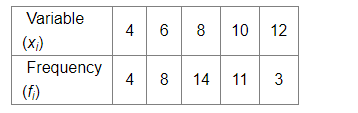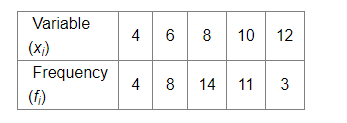# Obtain the mean of the following distribution:

Question:

Obtain the mean of the following distribution:Solution:

We know that,

Mean $=\frac{\sum x_{i} f_{i}}{\sum f_{i}}$

For the following data:Mean $=\frac{(4 \times 4)+(6 \times 8)+(8 \times 14)+(10 \times 11)+(12 \times 3)}{4+8+14+11+3}$

$=\frac{16+48+112+110+36}{40}$

$=\frac{322}{40}$

$=8.05$

Hence, the mean of the following distribution is 8.05 .